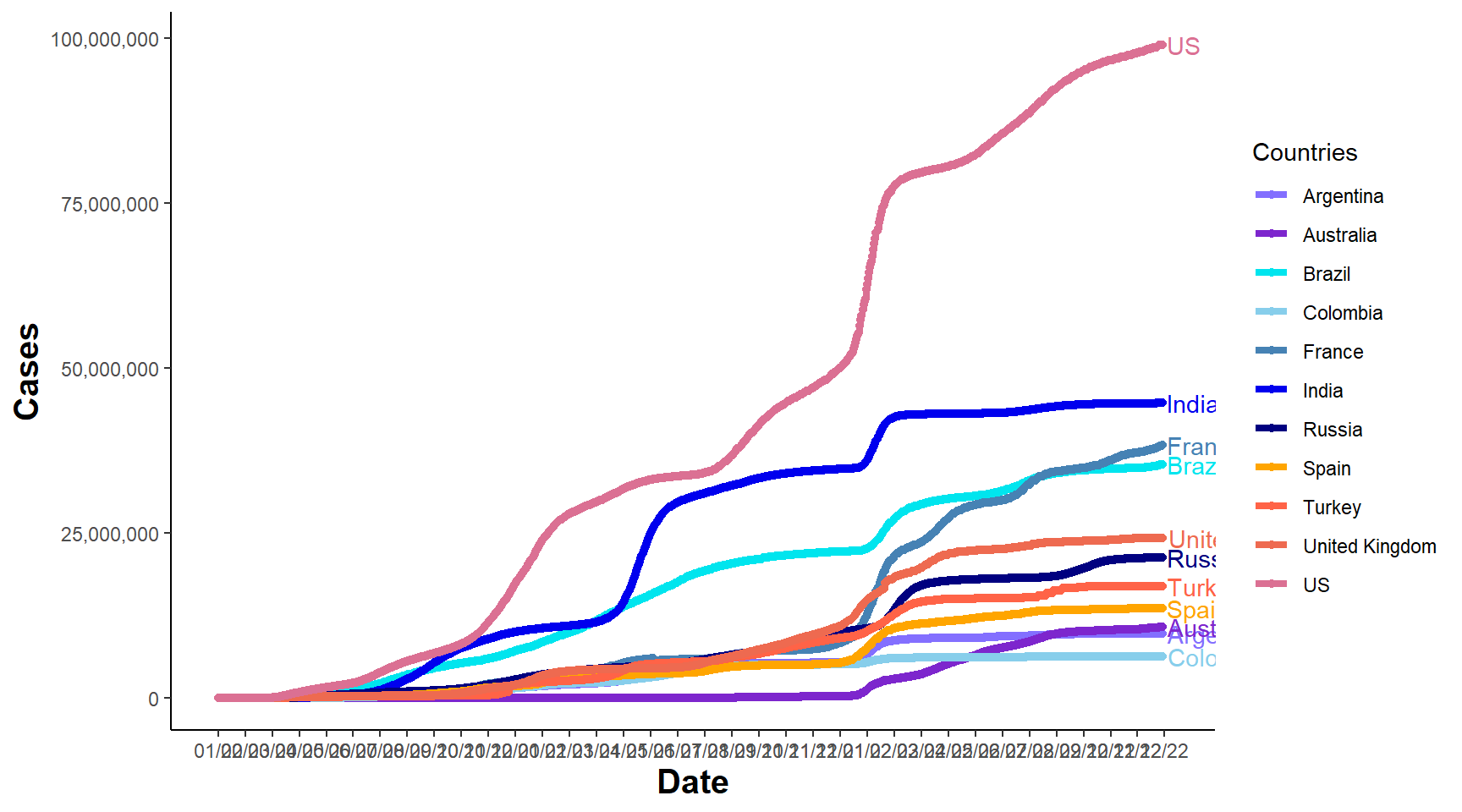## 6.1 Interactive Plots using Plotly

• Here we will use the COVID-19 data provided by John Hopkins University
library(ggplot2)
library(maps)
library(ggthemes)
library(plotly)
library(scales)
library(dplyr)
library(tidyr)

check.names = FALSE)
# head(d1)
• Data is in wide format let’s convert in long format for visualisation
# rename Provice/State and Country columns
colnames(d1)[1:2] = c("State", "Country")

d1.2 = pivot_longer(d1, cols = -c(State, Country, Lat, Long), names_to = "Date",
values_to = "Cases")
# convert dates

d1.2$Date = as.Date(d1.2$Date, format = "%m/%e/%y")
• Aggregate cases by day (dropping the state)
d2 = aggregate(d1.2$Cases, by = list(Lat = d1.2$Lat, Long = d1.2$Long, Country = d1.2$Country,
Date = d1.2$Date), FUN = sum) colnames(d2) = "Cases" # reorder d2 = d2[, c(4, 1, 2, 3, 5)] • Let’s find top 10 by case numbers • Using aggregate to find sum by country to find top 10 top1 = aggregate(d2$Cases, by = list(Date = d2$Date, Country = d2$Country), FUN = sum)
# select the last date to get overall total
top10 = top1[top1$Date == "2021-08-11", ] # select top 10 top10 = top10[order(-top10$x), ][1:10, ]
# let's include Aus
top10_country = c(top10$Country, "Australia") • Use ggplot to create a line chart 6.1 colnames(top1) = "Cases" data_p = top1[top1$Country %in% c(as.character(top10_country)), ]

p1 = ggplot(data = data_p, aes(Date, log(Cases), color = Country, group = Country)) +
geom_line(stat = "identity", size = 1) + scale_x_date(labels = date_format("%m/%y"),
breaks = "2 months") + theme_wsj()
p1Figure 6.1: Line Chart with Custom Theme

• Create custom color vector and a line chart with basic theme to convert to plotly 6.2
myCol2 = c("slateblue1", "purple3", "turquoise2", "skyblue", "steelblue", "blue2",
"navyblue", "orange", "tomato", "coral2", "palevioletred", "violetred", "red2",
"springgreen2", "yellowgreen", "palegreen4", "wheat2", "tan", "tan2", "tan3",
"brown", "grey70", "grey50", "grey30")

p2 = ggplot(data_p, aes(Date, Cases, group = Country, color = Country)) + geom_line(size = 1.5) +
geom_point(size = 1.5) + scale_colour_manual(values = myCol2, "Countries") +
geom_text(data = data_p[data_p$Date == max(data_p$Date), ], aes(x = as.Date(max(data_p$Date) + 4), label = Country), hjust = -0.01, nudge_y = 0.01, show.legend = FALSE) + expand_limits(x = as.Date(c(min(data_p$Date), max(data_p$Date) + 5))) + scale_x_date(breaks = seq(as.Date(min(data_p$Date)),
as.Date(max(data_p\$Date) + 5), by = "30 days"), date_labels = "%m/%y") + scale_y_continuous(labels = comma) +
theme_classic() + theme(axis.title = element_text(size = 15, face = "bold"))
p2Figure 6.2: Line Chart

• Convert to plotly for interactive graphics 6.3
fig_p2 = ggplotly(p2)
fig_p2

Figure 6.3: Interactive Line Chart